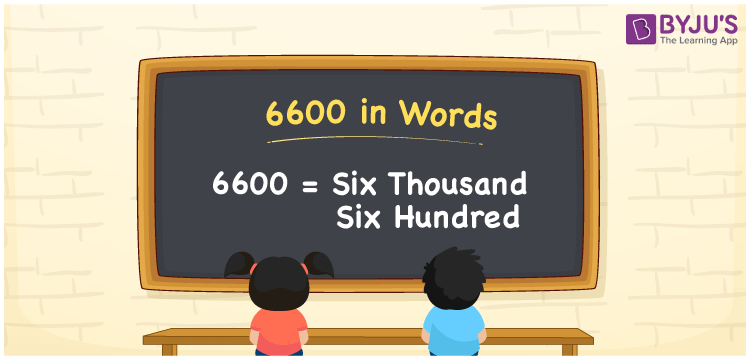6600 in words is written as “Six Thousand Six Hundred”. In Maths, 6600 is a cardinal number that expresses a quantity or value. For example, Meeta helped her friend by giving Rupees Six Thousand Six Hundred. Learn more about Numbers In Words and writing the number names in English at BYJU’S.

 6600 in Words Six Thousand Six Hundred Six Thousand Six Hundred in Numbers 6600

## 6600 in English Words

The name of number 6600 in English is given by:## How to Write 6600 in Words?

The number 6600 in words can be written using a place value system, where we can identify the position of each digit of the original number. Since 6600 is a four-digit number, thus,

 Thousands Hundreds Tens Ones 6 6 0 0

From the above table,

6 → Thousands

6 → Hundreds

0 → Tens

0 → Ones

Hence, when we read the number from right to left, it is given by Six Thousand Six Hundred.

### Expanded Form of 6600

We can write the expanded form as:

6 x Thousand + 6 × Hundred + 0 × Ten + 0 × One

= 6 x 1000 + 6 × 100 + 0 × 10 + 0 × 1

= 6000 + 600 + 0 + 0

= Six Thousand Six Hundred

6600 is a whole number that is succeeded by 6599 and preceded by 6601. Learn more about the number 6600 below:

• 6600 in Words – Six Thousand Six Hundred
• Is 6600 an odd number? – No
• Is 6600 an even number? – Yes
• Is 6600 a perfect square number? – No
• Is 6600 a perfect cube number? – No
• Is 6600 a prime number? – No
• Is 6600 a composite number? – Yes

## Frequently Asked Questions on 6600 in words

Q1

### What is 6600 in words?

6600 in words is given by Six Thousand Six Hundred.
Q2

### What is the rule to write 6600 in words?

To write the 6600 in words, we should use place value rule, where the position of each digit determined the number name.

6 → Thousands

6 → Hundreds

0 → Tens

0 → Ones

Hence, 6600 is written as Six Thousand Six Hundred, in words.

Q3

### What is the value of 6600 + 400 in words?

6600 + 400 = 7000, i.e., Seven Thousand in words.